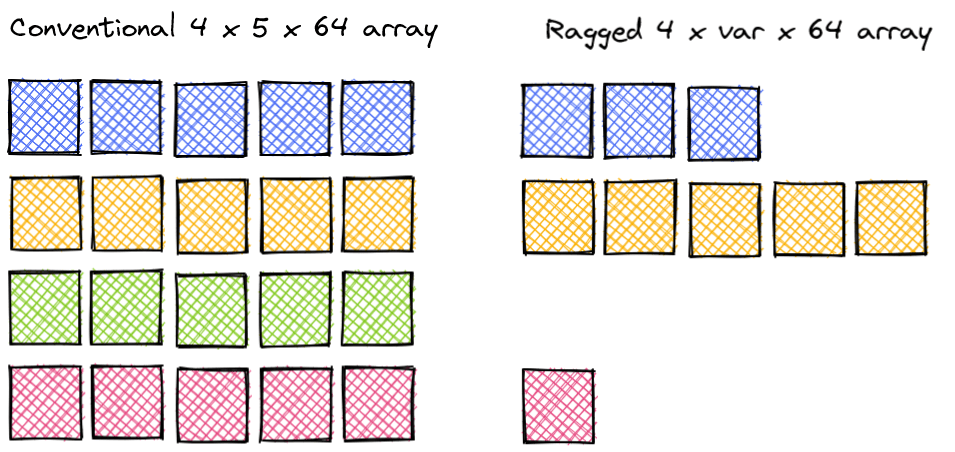## ragged-buffer

Efficient RaggedBuffer datatype that implements 3D arrays with variable-length 2nd dimension

### 9 releases

 0.4.8 May 2, 2023 Apr 30, 2023 Jul 28, 2022 Apr 11, 2022 Mar 31, 2022

#176 in Algorithms

Used in entity-gym-rs

MIT/Apache

77KB
2K SLoC

# ENN Ragged Buffer

This Python package implements an efficient `RaggedBuffer` datatype that is similar to a 3D numpy array, but which allows for variable sequence length in the second dimension. It was created primarily for use in enn-trainer and currently only supports a small selection of the numpy array methods.## User Guide

Install the package with `pip install ragged-buffer`. The package currently supports three `RaggedBuffer` variants, `RaggedBufferF32`, `RaggedBufferI64`, and `RaggedBufferBool`.

### Creating a RaggedBuffer

There are three ways to create a `RaggedBuffer`:

• `RaggedBufferF32(features: int)` creates an empty `RaggedBuffer` with the specified number of features.
• `RaggedBufferF32.from_flattened(flattened: np.ndarray, lenghts: np.ndarray)` creates a `RaggedBuffer` from a flattened 2D numpy array and a 1D numpy array of lengths.
• `RaggedBufferF32.from_array` creates a `RaggedBuffer` (with equal sequence lenghts) from a 3D numpy array.

Creating an empty buffer and pushing each row:

``````import numpy as np
from ragged_buffer import RaggedBufferF32

# Create an empty RaggedBuffer with a feature size of 3
buffer = RaggedBufferF32(3)
# Push sequences with 3, 5, 0, and 1 elements
buffer.push(np.array([[1, 2, 3], [4, 5, 6], [7, 8, 9]], dtype=np.float32))
buffer.push(np.array([[10, 11, 12], [13, 14, 15], [16, 17, 18], [19, 20, 21], [22, 23, 24]], dtype=np.float32))
buffer.push(np.array([], dtype=np.float32))  # Alternative: `buffer.push_empty()`
buffer.push(np.array([[25, 25, 27]], dtype=np.float32))
``````

Creating a RaggedBuffer from a flat 2D numpy array which combines the first and second dimension, and an array of sequence lengths:

``````import numpy as np
from ragged_buffer import RaggedBufferF32

buffer = RaggedBufferF32.from_flattened(
np.array([[1, 2, 3], [4, 5, 6], [7, 8, 9], [10, 11, 12], [13, 14, 15], [16, 17, 18], [19, 20, 21], [22, 23, 24], [25, 25, 27]], dtype=np.float32),
np.array([3, 5, 0, 1], dtype=np.int64))
)
``````

Creating a RaggedBuffer from a 3D numpy array (all sequences have the same length):

``````import numpy as np
from ragged_buffer import RaggedBufferF32

buffer = RaggedBufferF32.from_array(np.zeros((4, 5, 3), dtype=np.float32))
``````

### Get size

The `size0`, `size1`, and `size2` methods return the number of sequences, the number of elements in a sequence, and the number of features respectively.

``````import numpy as np
from ragged_buffer import RaggedBufferF32

buffer = RaggedBufferF32.from_flattened(
np.zeros((9, 64), dtype=np.float32),
np.array([3, 5, 0, 1], dtype=np.int64))
)

# Get size of the first/batch dimension.
assert buffer.size0() == 10
# Get size of individual sequences.
assert buffer.size1(1) == 5
assert buffer.size1(2) == 0
# Get size of the last/feature dimension.
assert buffer.size2() == 64
``````

### Convert to numpy array

`as_aray` converts a `RaggedBuffer` to a flat 2D numpy array that combines the first and second dimension.

``````import numpy as np
from ragged_buffer import RaggedBufferI64

buffer = RaggedBufferI64(1)
buffer.push(np.array([, , ], dtype=np.int64))
buffer.push(np.array([, ], dtype=np.int64))
assert np.all(buffer.as_array(), np.array([, , , , ], dtype=np.int64))
``````

### Indexing

You can index a `RaggedBuffer` with a single integer (returning a `RaggedBuffer` with a single sequence), or with a numpy array of integers selecting/permuting multiple sequences.

``````import numpy as np
from ragged_buffer import RaggedBufferF32

# Create a new `RaggedBufferF32`
buffer = RaggedBufferF32.from_flattened(
np.arange(0, 40, dtype=np.float32).reshape(10, 4),
np.array([3, 5, 0, 1], dtype=np.int64)
)

# Retrieve the first sequence.
assert np.all(
buffer.as_array() ==
np.array([[0, 1, 2, 3], [4, 5, 6, 7], [8, 9, 10, 11]], dtype=np.float32)
)

# Get a RaggedBatch with 2 randomly selected sequences.
buffer[np.random.permutation(4)[:2]]
``````

You can add two `RaggedBuffer`s with the `+` operator if they have the same number of sequences, sequence lengths, and features. You can also add a `RaggedBuffer` where all sequences have a length of 1 to a `RaggedBuffer` with variable length sequences, broadcasting along each sequence.

``````import numpy as np
from ragged_buffer import RaggedBufferF32

# Create ragged buffer with dimensions (3, [1, 3, 2], 1)
rb3 = RaggedBufferI64(1)
rb3.push(np.array([], dtype=np.int64))
rb3.push(np.array([, , ], dtype=np.int64))
rb3.push(np.array([, ], dtype=np.int64))

# Create ragged buffer with dimensions (3, [1, 1, 1], 1)
rb4 = RaggedBufferI64.from_array(np.array([0, 3, 10], dtype=np.int64).reshape(3, 1, 1))

rb5 = rb3 + rb4
assert np.all(
rb5.as_array() == np.array([, , , , , ], dtype=np.int64)
)
``````

### Concatenation

The `extend` method can be used to mutate a `RaggedBuffer` by appending another `RaggedBuffer` to it.

``````import numpy as np
from ragged_buffer import RaggedBufferF32

rb1 = RaggedBufferF32.from_array(np.zeros((4, 5, 3), dtype=np.float32))
rb2 = RaggedBufferF32.from_array(np.zeros((2, 5, 3), dtype=np.float32))
rb1.extend(r2)
assert rb1.size0() == 6
``````

### Clear

The `clear` method removes all elements from a `RaggedBuffer` without deallocating the underlying memory.

``````import numpy as np
from ragged_buffer import RaggedBufferF32

rb = RaggedBufferF32.from_array(np.zeros((4, 5, 3), dtype=np.float32))
rb.clear()
assert rb.size0() == 0
``````